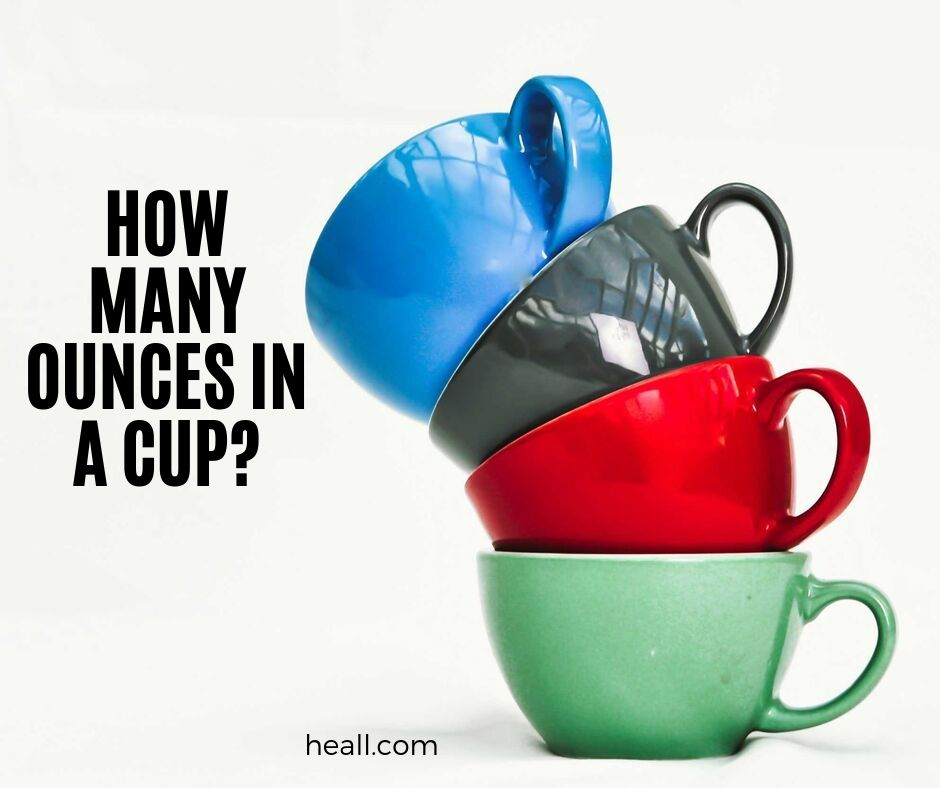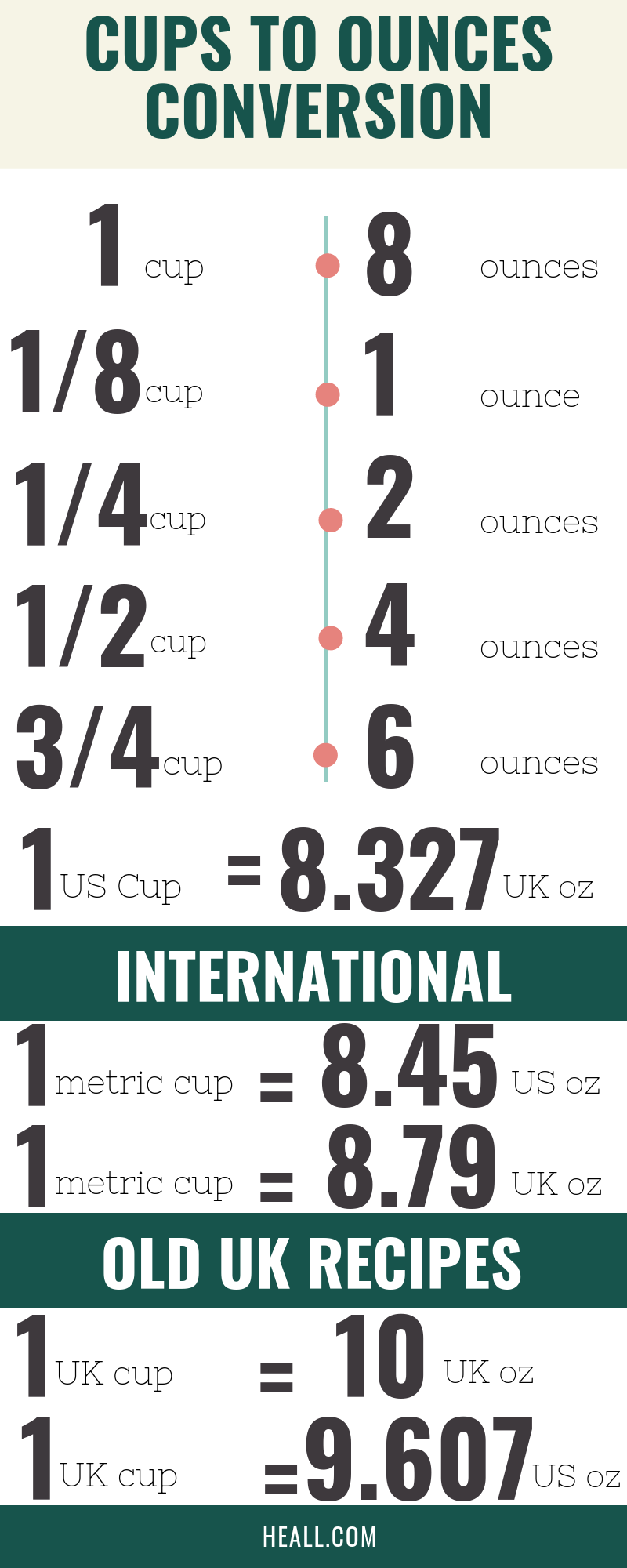# How Many Ounces In A Cup?

If you have never thought that metric conversions could be a problem once in your entire life, then you have to think again. When you drink coffee in the morning, we always refer to the act of doing so as drinking a cup. But how much is a cup of coffee?

Does one cup of coffee equate to the standard measurement of cup the measurement unit? What if you prefer ounces instead of cups? What is the difference between an ounce and a fluid ounce? Specifically, how many ounces are in a cup?The first thing to breakdown here is which cup are we referring to? The cup as in where the liquid beverage is poured on or the cup in the metric system? This is important because a standard cup, the one we use to pour on the coffee or tea is six ounces while the cup that is used as a standard of measurement has no universal conversion to ounces.

Now, why is there a need to measure cups to ounces and vice versa? According to Cooking Light, the main reason behind this is cooking. Cup is the standard cooking measurement. All recipes in cookbooks are listed and measured through cups.

However, when the typical baker or cook goes into the supermarket to buy liquid ingredients for food, the standard packaging measurement is ounces. This is the reason why we should know how many ounces are present in a cup.

## What is a cup measurement in UK?

We have said a while back that cup to ounce conversion can get a little tricky because it actually depends on where you are in the world. There exists an international metric cup but for countries such as the United States, the UK, Japan and Canada, cups to ounces can be a little different.

For example, in Canada, one cup is equal to 7.6 ounces while one cup in Japan is only 6.7. Of course, the famous cup to ounce discrepancy is from the US and the UK. For example, one US cup is equal to 8 US fluid ounces but 1 US cup is equal to 8.327 UK fluid ounces.

1 Canadian cup = 7.6 ounces

1 Japanese cup = 6.7 ounces

1 US cup = 8 ounces

1 US cup = 8.327 UK fluid ounces

But for specific cases where a particular measuring tool, for example in baking, was manufactured in either of the mentioned countries and you want to get an accurate measurement for it, you will need to be familiar with the specific cup measurements for each country.

So back to the question at hand. What is a cup measurement in the UK?

one cup in the US is equal to 8 US fluid ounces while one cup in the UK is 10 UK fluid ounces. Why is this so?

This is because in the UK, weights are measured and not volumes. Thus, the UK is more familiar with the ounce and not the cup. But since most recipe books are now using cups, they needed to convert their ounces into cups.

1 UK cup = 10 UK fluid ounces

However, one cup in the UK is 284.13mL while one cup in the US is 236.59mL, that is why the weight conversion to ounces slightly varies. So if you are in the US but your recipe book uses the UK conversion, might as well remove two tablespoons from your one cup. But whether you adjust it or not, there will be no difference in the dish so basically, it is your call.

## What is fluid ounce?

Ounces are different from fluid ounces because of one reason only; ounces measure weight while fluid ounces measure volume. Fluid ounces, as the term implies, are used to weight the volume of liquid substances. Thus, ounces and fluid ounces are not interchangeable terms because they measure two different things.

For example, a baker knows that a bag of flour can be converted to ounces but not into fluid ounces. In the same manner, when a bartender is asked to give eight ounces of malt beer, he will not be confused because when it comes to beverages, ounce means fluid ounces.

Fun fact, the normal cup measures to approximately eight ounces in weight and eight fluid ounces in volume. In this case, ounces and fluid ounces can be used in one conversion because they measure different things.

Moreover, net weight ounces should not also be confused with fluid ounces.

When the label of your liquid ingredient is net weight ounces, it is expressed in ounces only but this rarely happens because net weight ounces are most commonly used in dry ingredients or dry products. The symbol for fluid ounce is fl.oz..

## How many cups in a fluid ounce?

The standard conversion of one cup is eight fluid ounces but what if we need to know how many cups are in a fluid ounce? According to Exploratorium, there are 1/4 cups (the exact computation is 0.125 where 8 multiplied to 0.125 is one) in a fluid ounce if we use the US conversion but slightly less than 1/4 cups (the exact computation is 0.12009) when the UK conversion is used.

1 fluid ounce = 0.125 cup

Now, we have already said that fluid ounces are only used to measure the volume of fluid ingredients. This is quite important because there are specific measuring tools for dry and liquid ingredients.

Thus, the measuring tools for dry ingredients may be labelled in ounces while the measuring tools for liquid ingredients are automatically in fluid ounces.

It is important to never overlook or be too complacent about just grabbing a measuring spoon just because they are all spoons because you might be getting the wrong measurements for your dish.

## How many cups is 1/4 oz?

1/4 oz = 0.03125 US cup

## How many cups is 2/3 oz?

2/3 oz = 0.083 US cup

## How many cups is 3/4 oz?

3/4 oz = 0.09375 US cup

## How many cups is 1.75 oz?

1.75 oz = 1.75/8 = 0.21875 US cup

## How many cups is 3.5 oz?

3.5 oz = 0.4375 US cup

## How many cups is 5.4 oz?

5.4 oz = 0.675 US cup

## How many cups is 6.5 oz?

6.5 oz = 0.8125 US cup

## How many cups is 7.5 oz?

7.5 oz = 0.9375 US cup

## Cups to ounces conversion table

If we follow the exact conversion of cups to ounces which 8.45 ounces in a cup in the US and 8.79 for the UK, the following conversions can be made:

US CupsOunce conversionUK CupsOunce Conversion
18.4518.79
216.9217.58
325.65326.37
434.1435.16
542.55543.95
1084.51087.9

If you also need a conversion table for cups to fluid ounces using the international metric system, this cup to fluid ounce conversion might come in handy:

1 Cup = 8 US Fluid Ounces

1 Cup = 10 UK Fluid Ounces

CupUK Fluid OunceUS Fluid Ounce
1/81.251
1/42.52
1/254
3/47.56
1108

You will need both of these tables if you are a pharmacist or a chemist. But in everyday life, you need these conversions if you are fond of baking or cooking. Being familiar with these will help you in your next visit to the supermarket because you will now become more aware of the accurate conversions for each.

Now that you know all of this, knowing how many ounces in a cup suddenly becomes a big deal, but an interesting one for that matter. Cups to ounces and cups to fluid ounces are not the only measurements that can get complicated.

Tablespoons to cups can be a bit of a trick to and like ounces, the standard measurement for it may also be complex at times. Nonetheless, if you have never thought that you would think about metric conversions this thorough once in your entire life, then the question on how many ounces in a cup for sure have made you rethink that.

Categories Q&A